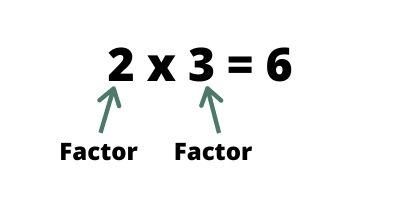# Factors of 28

## What is the factor?

Factors are integers you multiply together to get another integer. Also remember that the factors always include 1 and itself.NOTE: When finding the factors of a number, ask yourself “what numbers can be multiplied together to give me this number?.

## How to find the factors of 28?

THINK: What pairs of numbers can be multiplied together to get 28?

Step 1: 1 x 28 = 28, so put these numbers in the factor list.

 1 … 28

Step 2: Take 2 and divide with 28. It gives 2 x 14 = 28.

 1 2 14 28
Step 3:Take 3 and divide with 28. The remainder will be 1. But factors always give 0 remainder. This means 3 is NOT a factor of 28.
Step 4: Take 4 and divide with 28. It gives 4 x 7 = 28.
 1 2 4 7 14 28
Step 5: Take 5 and divide with 28. The remainder will be 3. But factors always give 0 remainder. This means 5 is NOT a factor of 28.
Step 6: Take 6 and divide with 28. The remainder will be 4. This means 6 is NOT a factor of 28.
Step 7: Take 7 and divide with 28. It gives 7 x 4 = 28 but factors 7 and 4 are already in the list. So you dont need to repeat the number.
 1 2 4 7 14 28
Step 8: Take 8 and divide with 28. The remainder will be 4. This means 8 is NOT a factor of 28.
Step 9: Take 9 and divide with 28. The remainder will be 1. This means 9 is NOT a factor of 28.

Step 10: Take 10 and divide with 28. The remainder will be 8. This means 10 is NOT a factor of 28.

So the factors of 28 are 1, 2, 4, 7, 14, and 28.

TIPS: Try to keep tables (at least till 20) on your finger tips. Revise tables every day## Is 28 a prime or composite number?

28 is a composite number not prime number because it has more than 2 factors.

What is composite number? – The composite numbers are the positive integers which have more than two factors.

What is prime number? – An integer number that is divisible by two distinct integers, 1 and itself.

## What is the prime factorization of 28

The prime factorization of 28 are  2 x 2 x 7 (i.e. 22 × 7)

## Factor pairs of 28

Factors are often given as pairs of numbers, which multiply together to give the original number. These are called factor pairs

What is factor pairs: Factor pairs are combinations of two factors that multiply together to give the original number.

So for 28, there are 6 factor pairs

1 x 28 = 28
2 x 14 = 28
4 x 7 = 28
7 x 4 = 28
14 x 2 = 28
28 x 1 = 28

## Is 28 a square number?

No, 28 is not a square number.
The square root of 28 is 5.292.
The square of 28 is 784.

Hope you have learned to solve the factors of 28.

Scroll to Top